Ex 15.1

Chapter 14 Class 10 Probability
Serial order wise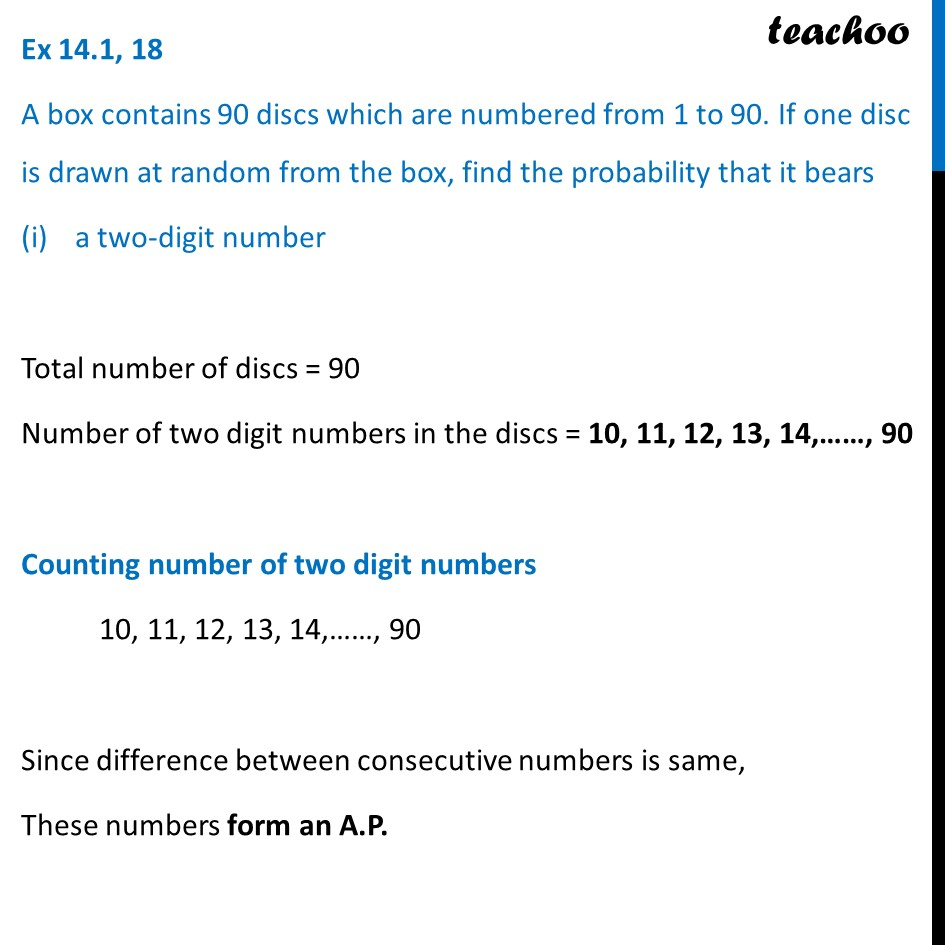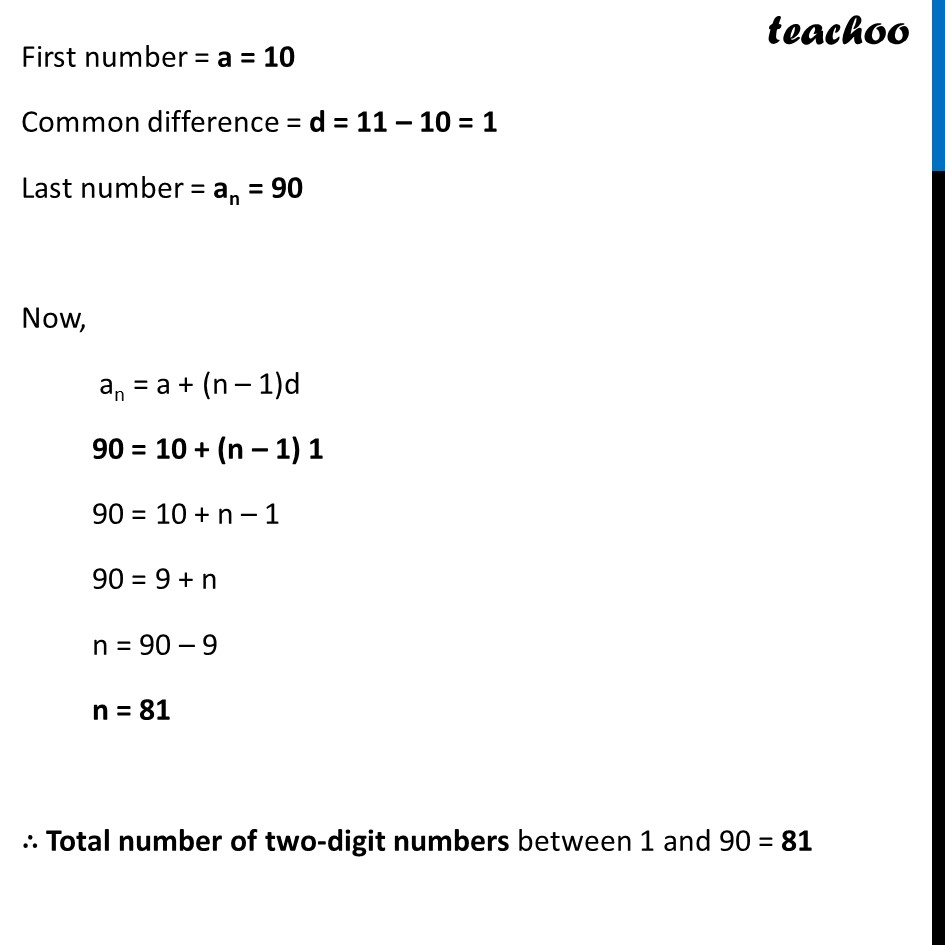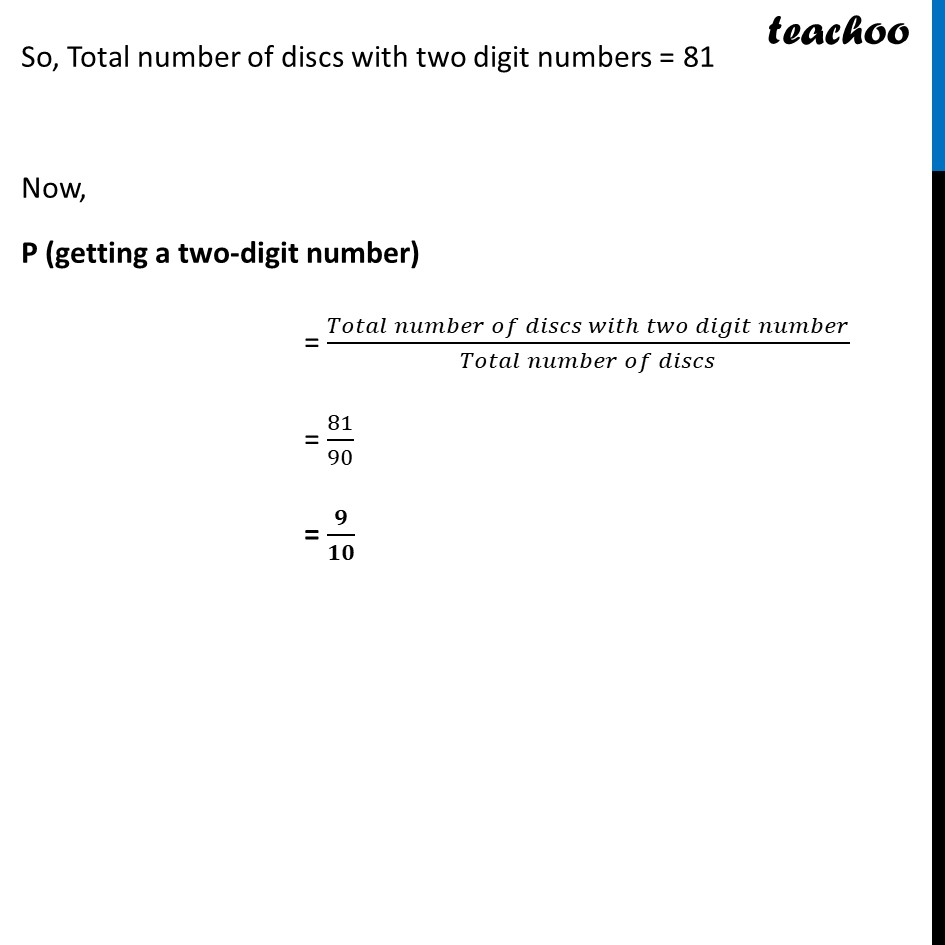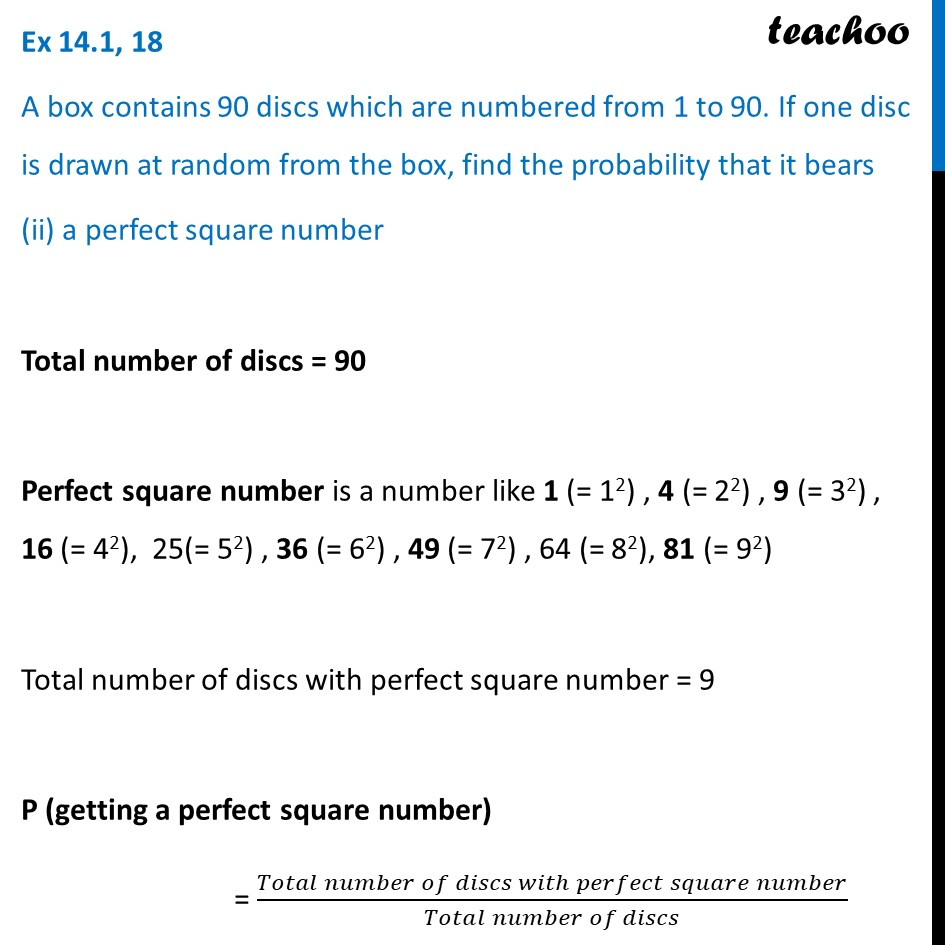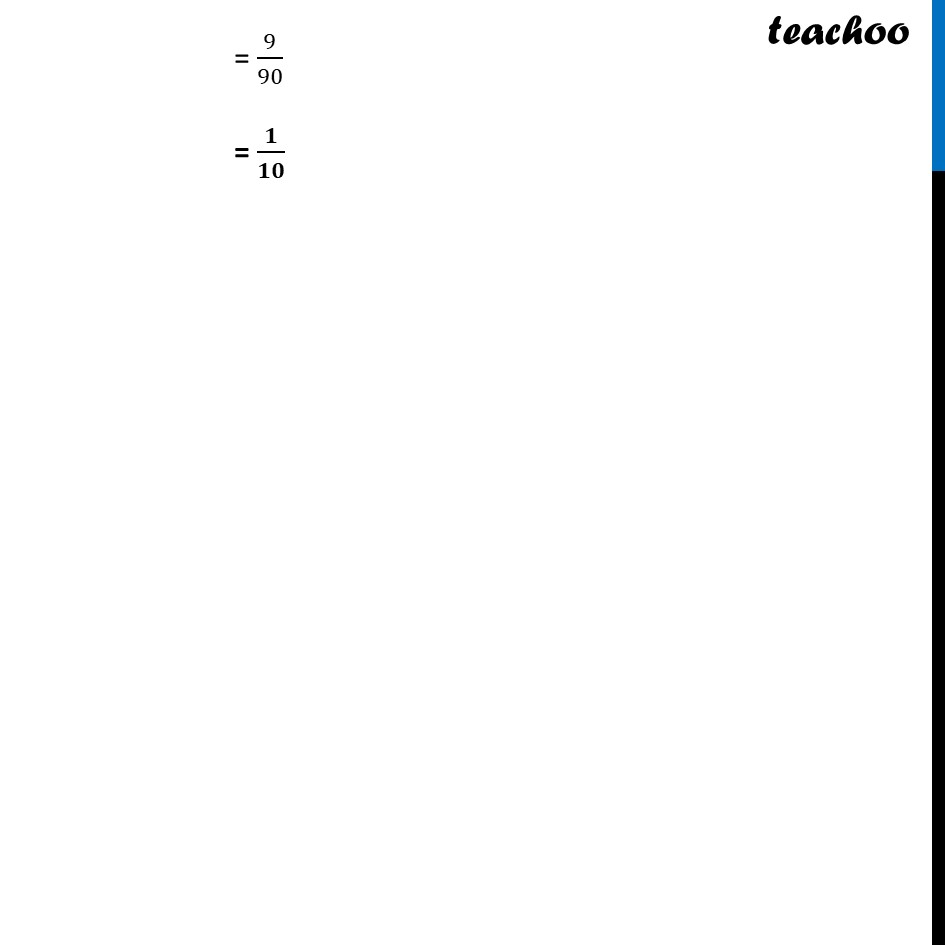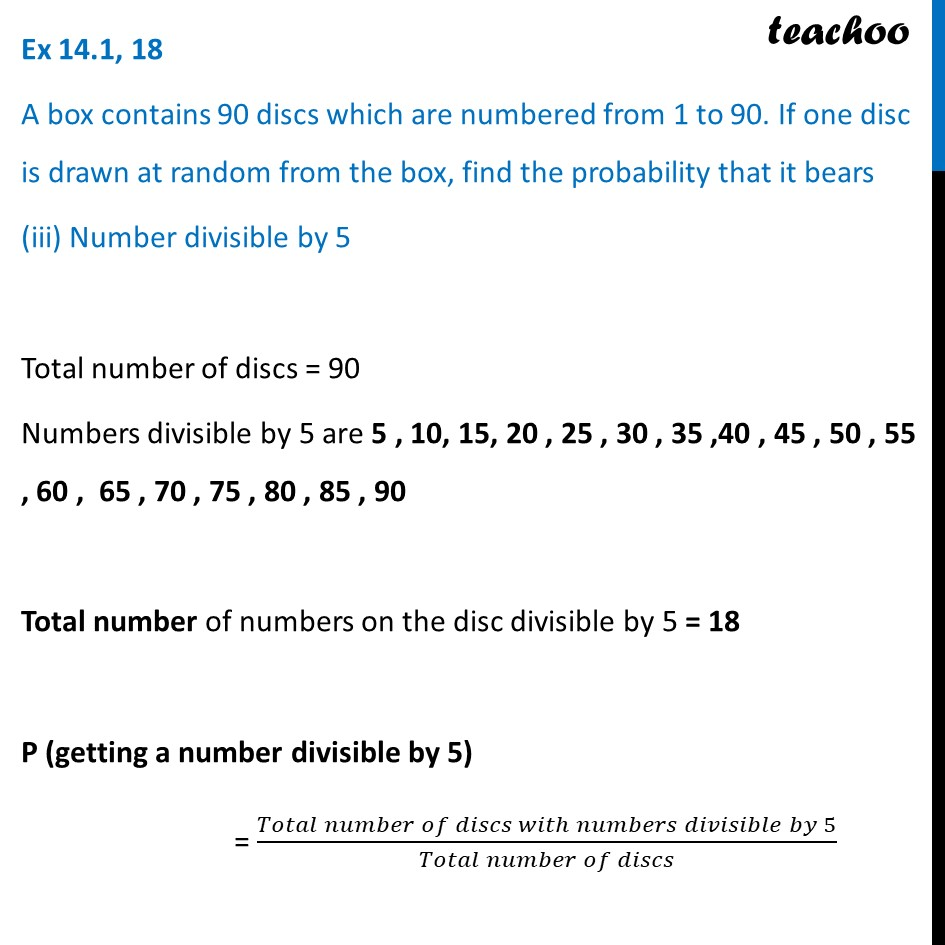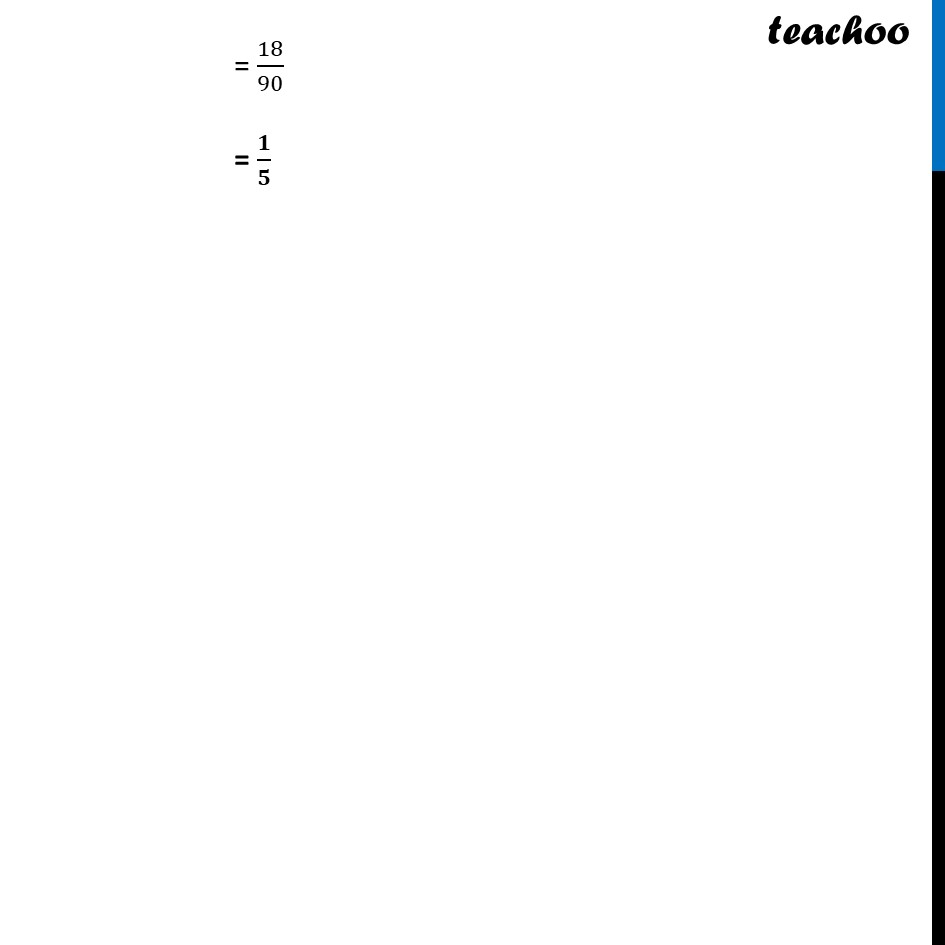Learn in your speed, with individual attention - Teachoo Maths 1-on-1 Class

### Transcript

Ex 14.1, 18 A box contains 90 discs which are numbered from 1 to 90. If one disc is drawn at random from the box, find the probability that it bears a two-digit number Total number of discs = 90 Number of two digit numbers in the discs = 10, 11, 12, 13, 14,……, 90 Counting number of two digit numbers 10, 11, 12, 13, 14,……, 90 Since difference between consecutive numbers is same, These numbers form an A.P. First number = a = 10 Common difference = d = 11 – 10 = 1 Last number = an = 90 Now, an = a + (n – 1)d 90 = 10 + (n – 1) 1 90 = 10 + n – 1 90 = 9 + n n = 90 – 9 n = 81 ∴ Total number of two-digit numbers between 1 and 90 = 81 So, Total number of discs with two digit numbers = 81 Now, P (getting a two-digit number) = (𝑇𝑜𝑡𝑎𝑙 𝑛𝑢𝑚𝑏𝑒𝑟 𝑜𝑓 𝑑𝑖𝑠𝑐𝑠 𝑤𝑖𝑡ℎ 𝑡𝑤𝑜 𝑑𝑖𝑔𝑖𝑡 𝑛𝑢𝑚𝑏𝑒𝑟)/(𝑇𝑜𝑡𝑎𝑙 𝑛𝑢𝑚𝑏𝑒𝑟 𝑜𝑓 𝑑𝑖𝑠𝑐𝑠) = 81/90 = 𝟗/𝟏𝟎 Ex 14.1, 18 A box contains 90 discs which are numbered from 1 to 90. If one disc is drawn at random from the box, find the probability that it bears (ii) a perfect square number Total number of discs = 90 Perfect square number is a number like 1 (= 12) , 4 (= 22) , 9 (= 32) , 16 (= 42), 25(= 52) , 36 (= 62) , 49 (= 72) , 64 (= 82), 81 (= 92) Total number of discs with perfect square number = 9 P (getting a perfect square number) = (𝑇𝑜𝑡𝑎𝑙 𝑛𝑢𝑚𝑏𝑒𝑟 𝑜𝑓 𝑑𝑖𝑠𝑐𝑠 𝑤𝑖𝑡ℎ 𝑝𝑒𝑟𝑓𝑒𝑐𝑡 𝑠𝑞𝑢𝑎𝑟𝑒 𝑛𝑢𝑚𝑏𝑒𝑟)/(𝑇𝑜𝑡𝑎𝑙 𝑛𝑢𝑚𝑏𝑒𝑟 𝑜𝑓 𝑑𝑖𝑠𝑐𝑠) = 9/90 = 𝟏/𝟏𝟎 Ex 14.1, 18 A box contains 90 discs which are numbered from 1 to 90. If one disc is drawn at random from the box, find the probability that it bears (iii) Number divisible by 5 Total number of discs = 90 Numbers divisible by 5 are 5 , 10, 15, 20 , 25 , 30 , 35 ,40 , 45 , 50 , 55 , 60 , 65 , 70 , 75 , 80 , 85 , 90 Total number of numbers on the disc divisible by 5 = 18 P (getting a number divisible by 5) = (𝑇𝑜𝑡𝑎𝑙 𝑛𝑢𝑚𝑏𝑒𝑟 𝑜𝑓 𝑑𝑖𝑠𝑐𝑠 𝑤𝑖𝑡ℎ 𝑛𝑢𝑚𝑏𝑒𝑟𝑠 𝑑𝑖𝑣𝑖𝑠𝑖𝑏𝑙𝑒 𝑏𝑦 5)/(𝑇𝑜𝑡𝑎𝑙 𝑛𝑢𝑚𝑏𝑒𝑟 𝑜𝑓 𝑑𝑖𝑠𝑐𝑠) = 18/90 = 𝟏/𝟓